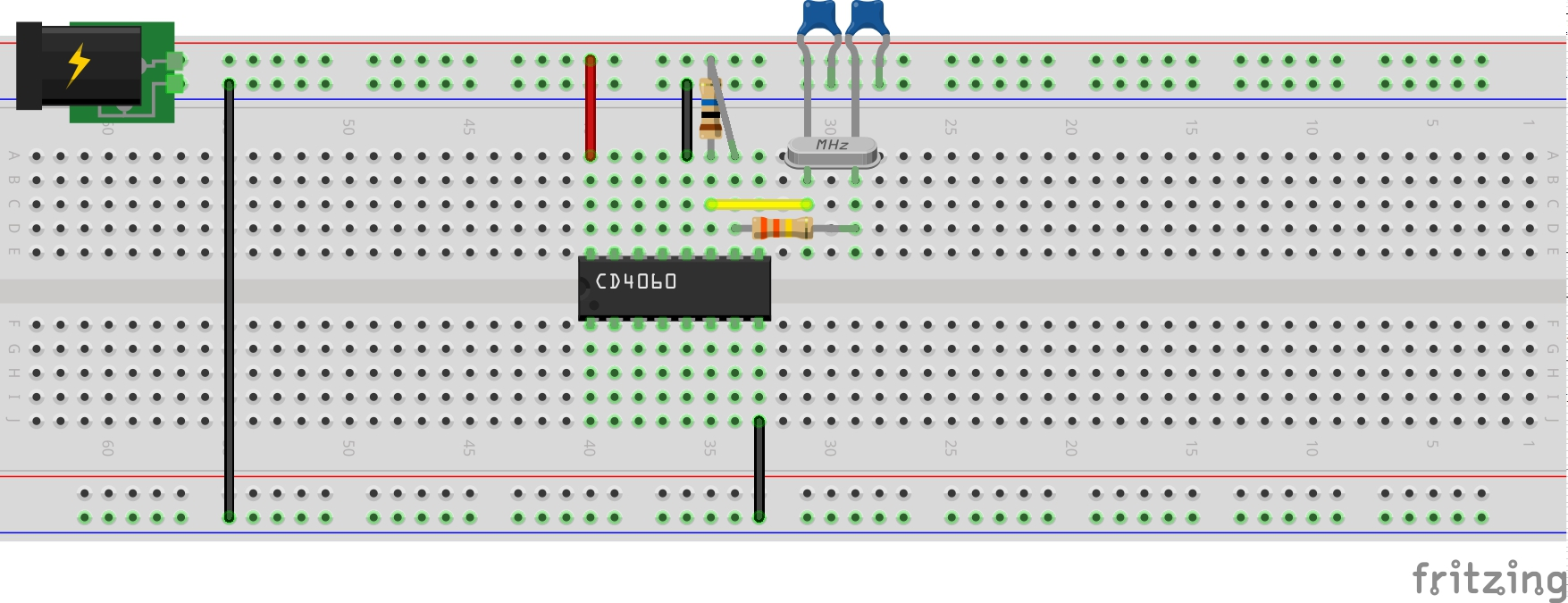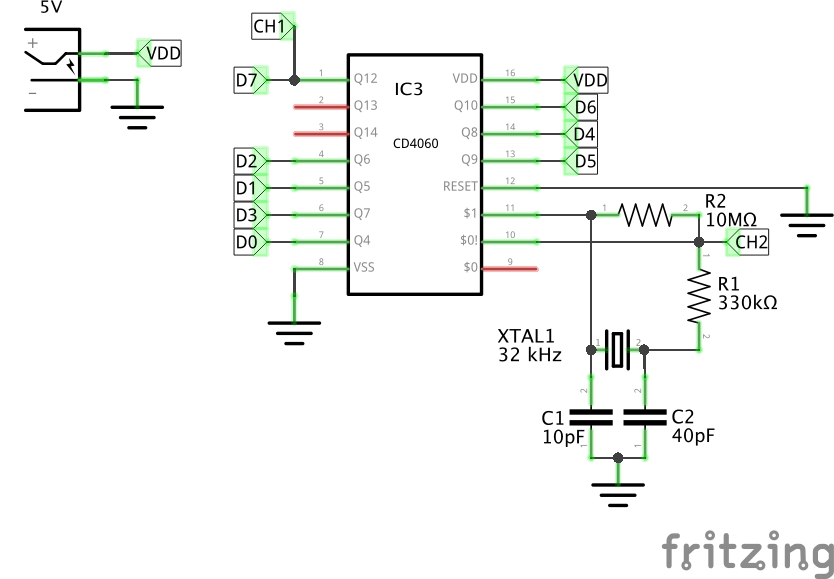# #294 CD4060/CrystalOscillator

Exploring the behaviour of the CD4060 ripple counter driven by a crystal oscillator.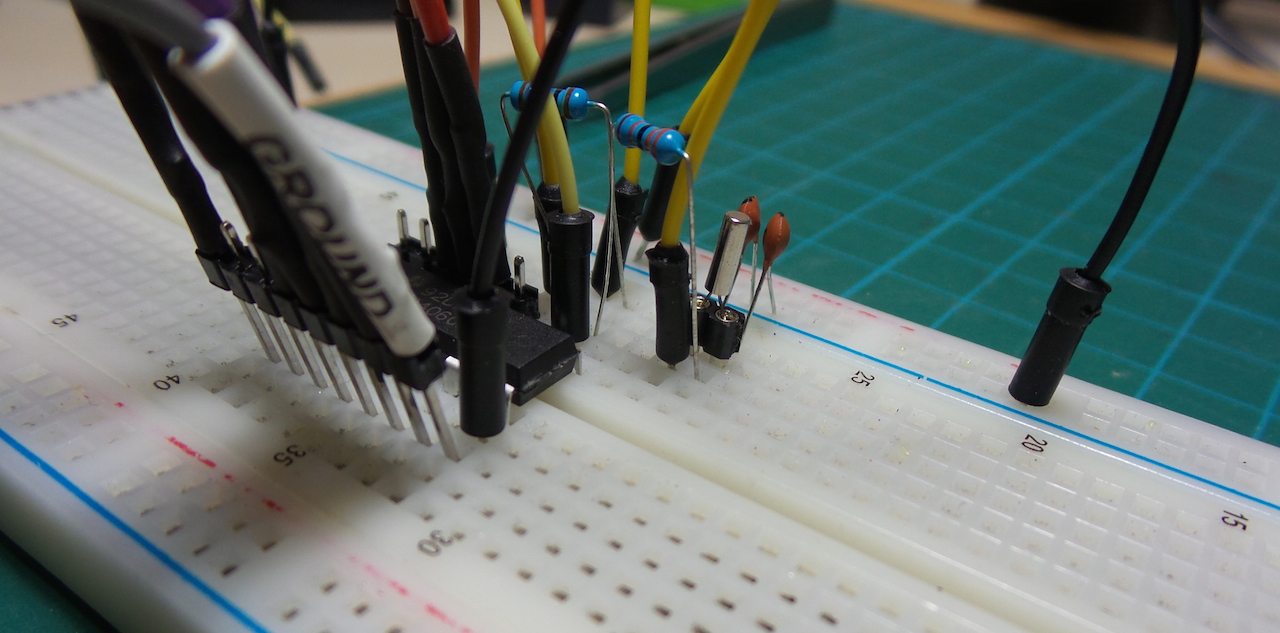The CD4060 datasheet describes a crystal oscillator configuration for self-driving the ripple counter. The use of a crystal provides very precise (but fixed) frequency control.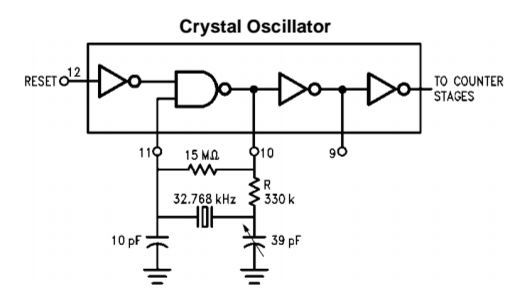See the LEAP#293 CD4060/RCOscillator project a similar circuit that is RC controlled, thus allows variable frequency control.

In this test I’m using a 32.768kHz crystal. The resulting oscillation looks like this (tapped at the net marked CH2 in the schematic):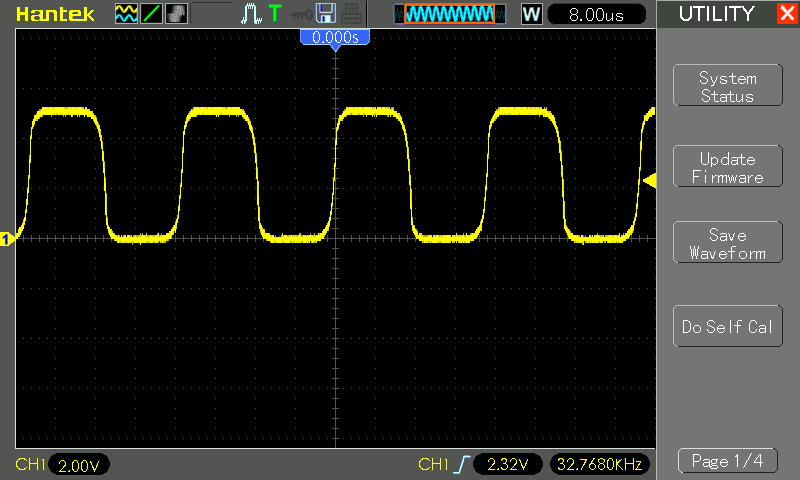### Ripple Counter

The CD4060 is a 14 stage ripple counter constructed of RS flip-flop units - see the functional diagram from the datasheet: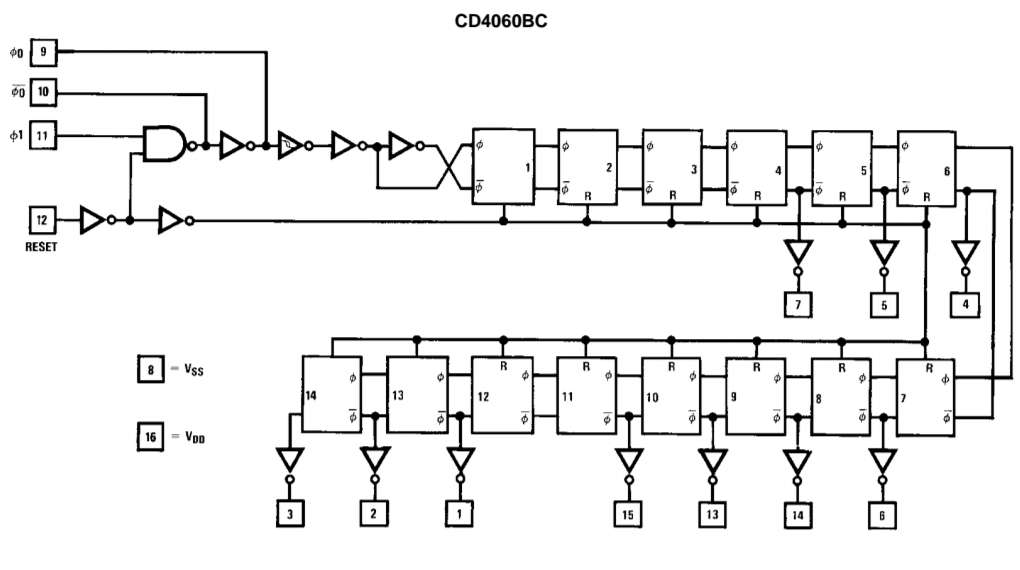The input signal passes 4 stages before the first output is tapped (Q4). Thus the first (Q4) output signal divides the input frequency by a factor of 2^4

Stage 11 (Q11) of the ripple counter is also not exposed on a pin.

The lack of Q0-3 and Q11 is I think just pin economics so it all fits it in a DIP16 package.

The performance is summarised in the following table and the logic analyzer (LA) capture. While 32.768kHz may sound like an odd crystal frequency, the table below gives away its purpose: the ripple counter divides this frequency into lots of familiar powers of 2! Also note how under crystal control, the actual frequency (as measured with an oscilloscope) is exactly equal to the theoretical frequency.

Signal LA Frequency (theory) Frequency (actual) Note
CH2   32768Hz 32768Hz f-input
Q4 07 2048Hz 2048Hz f-input/2^4
Q5 06 1024Hz 1024Hz f-input/2^5
Q6 05 512Hz 512Hz f-input/2^6
Q7 04 256Hz 256Hz f-input/2^7
Q8 03 128Hz 128Hz f-input/2^8
Q9 02 64Hz 64Hz f-input/2^9
Q10 01 32Hz 32Hz f-input/2^10
Q12 00 8Hz 8Hz f-input/2^12
Q13   4Hz 4Hz f-input/2^13
Q14   2Hz 2Hz f-input/2^14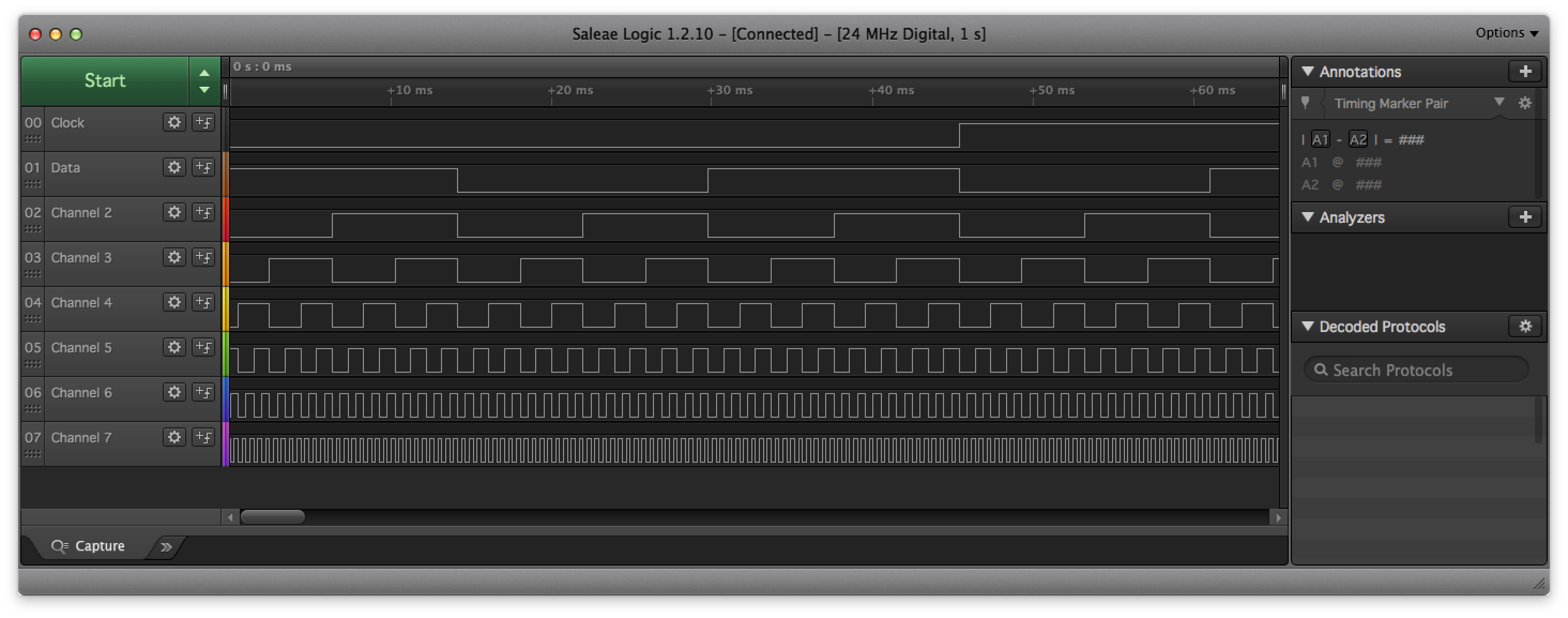## Construction